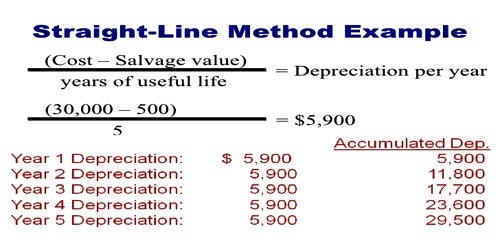Accounting

# Straight Line Method for Calculating DepreciationStraight Line Method or Fixed Installment Method for Calculating Depreciation

Depreciation is a decrease in the price of an asset with the course of time, due in particular to wear and tear. All assets whose advantage is derived for a long period of time, generally more than one year period are called as Fixed Assets.

Under Straight Line Method, the same amount of depreciation is charged every year throughout the life of the asset. The amount and rate of depreciation are calculated as under.

1. Amount of depreciation = (Total cost – Scrap value) / Estimated Life
2. Rate of depreciation = (Amount of Depreciation / Original Cost) x 100

Example: A company purchased Machinery for Rs.1,00,000. Its installation costs amounted to Rs.10,000. It’s estimated life is 5 years and the scrap value is Rs.5,000. Calculate the amount and rate of depreciation

Solution:

Total cost = Purchase Price + Installation Charges

= Rs.1,00,000 + Rs.10,000 = Rs. 1,10,000

Amount of depreciation = (Total cost – Scrap value) / Estimated Life

(Rs.1,10,000 – Rs.5,000) / 5 = Rs.21,000

Rate of depreciation = (Amount of Depreciation / Original Cost) x 100

= (Rs.21,000 / Rs.1,10,000) x 100 =  19.09%

Note: Under straight line method, for each of the five years, the amount of depreciation to be charged will be Rs.21,000.

Merits:

• Simplicity: It is very simple and easy to understand.
• Easy to calculate: It is easy to calculate the amount and rate of depreciation.
• Assets can be completely written off: Under this method, the book value of the asset becomes zero or equal to its scrap value at the expiry of its useful life.

Demerits:

The amount of depreciation is same in all the years, although the usefulness of the machine to the business is more in the initial years than in the later years.